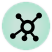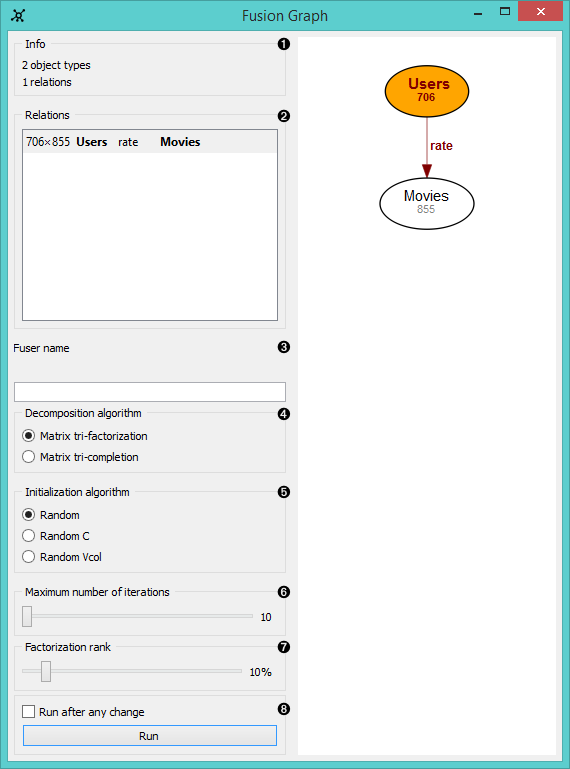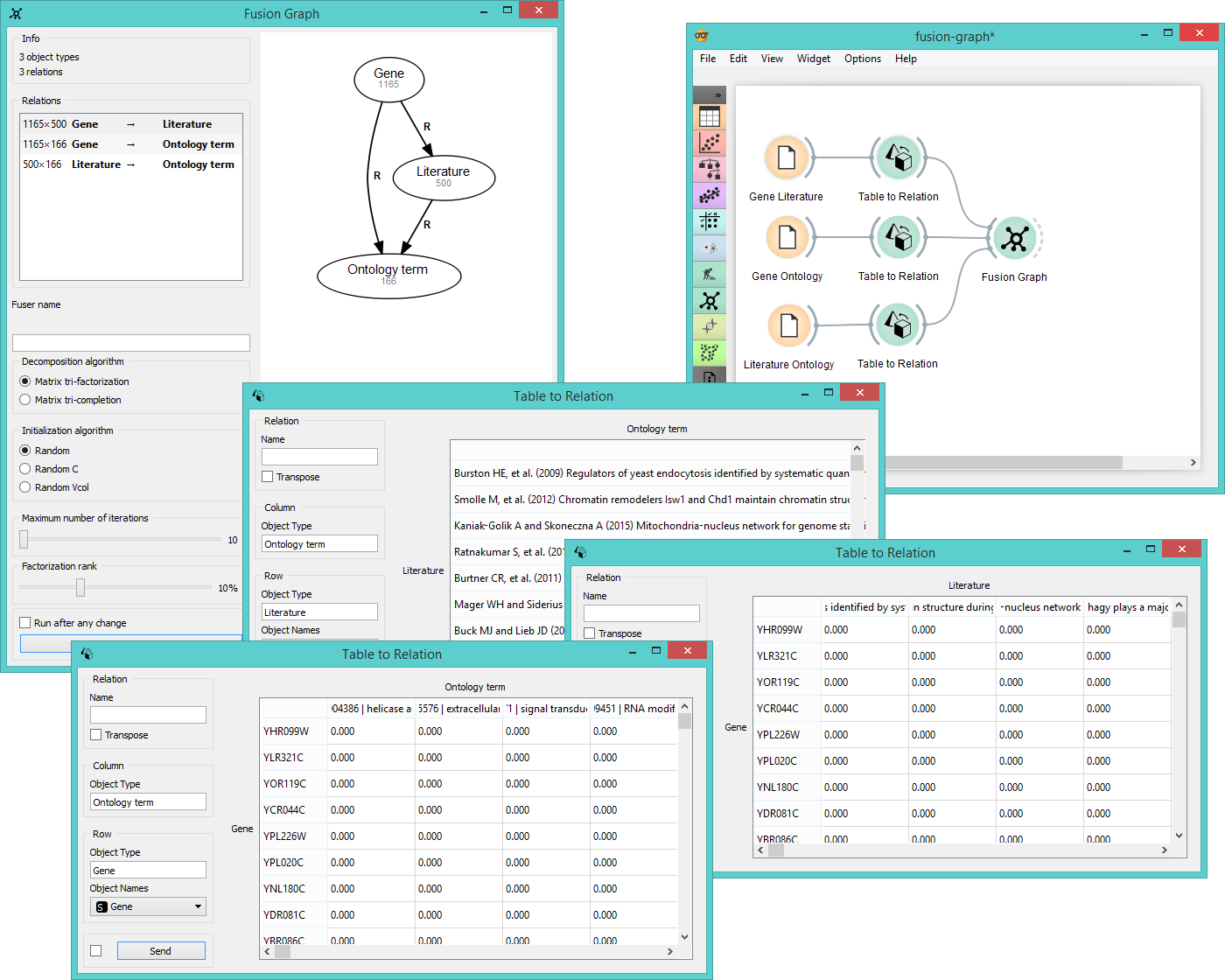# Fusion Graph¶Constructs a data fusion graph and runs collective matrix factorization algorithm.

## Signals¶

Inputs:

• Relation

Relationships between two groups of objects.

Outputs:

• Relation

Relationships between two groups of objects.

• Fitted Fusion Graph

Fitted collective latent data model.

• Fusion Graph

Input data system.

## Description¶

Fusion Graph widget performs data fusion by collective matrix factorization. It fuses multiple related data sets into one comprehensive structure. The widget returns a relational structure of the entire data system estimated by a collective latent factor approach.1. Information on the input (object types are nodes, relations are links between the nodes).
2. List of identified relations. Click on the relation to output it.
3. Specify a descriptive name for your fusion system.
4. Select the algorithm for factorization:
• matrix tri-factorization decomposes each relation matrix into three latent matrices and shares the latent matrices between related data sets. Unknown values are imputed prior to collective factorization.
• matrix tri-completion works the same as matrix tri-factorization, but does not require relation matrices to be fully observed.
5. Select the initialization algorithm for matrix factorization.
6. Set the maximum number of iterations used for factorization. Default is 10.
7. Set the factorization rank (the ratio of data compression based on the input data). Default is 10%.
8. If Run after every change is ticked, the widget will automatically commit changes. Alternatively press Run. For large data sets we recommend to commit the changes manually.

## Example¶

The example below shows how to fuse several data sets together. Say we have the data on ontology terms for many genes, literature on ontology terms and literature on genes. To fuse these data together we first use Table to Relation widget, where we manually set the object type and relation names. Fusion Graph will compile the fusion graph of our three data sets with connections between object types based on previously defined data relations, display the connections and run matrix decomposition algorithm.